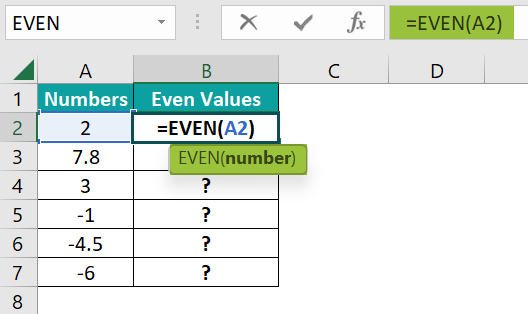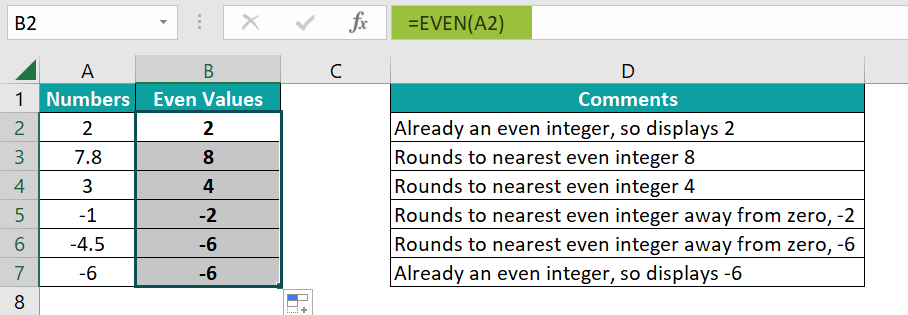# EVEN Function in ExcelArticle byExcelMojo Team## What is EVEN Function in Excel?

The Excel EVEN function rounds up numbers to the next even integer away from zero. Therefore, in the case of positive numbers, they become larger, while the negative ones are rounded to a more negative number. For instance, =EVEN(3) returns 4 while =EVEN(-3) returns -4.

Consider a few numbers below in Column A, which can be rounded off to the nearest even integer. Enter the formula =EVEN(A2) in cell B2. Press Enter and drag the Autofill handle up to B5. You observe that the numbers have been rounded off to the next even integer away from zero.

###### Key Takeaways
• The Excel EVEN function returns the next even integer of a number supplied to it as an argument. The positive and negative numbers it returns move further from zero.
• This function belongs to the class of Math and Trig functions. It takes a single argument. Only numeric values can be given as arguments; else, you get an error.
• This function is helpful when performing calculations for items in pairs or those involving counting in twos.

### EVEN() Excel Formula

The syntax for the EVEN() function in Excel is as follows:

Arguments

• number  (mandatory) –  The number to round to the nearest even integer from zero.

### How to Use EVEN Excel Function?

Now, we can find the nearest even integer in Excel in the following ways. The two ways include:

• Manually typing the Excel EVEN function in an Excel cell
• Selecting the EVEN() formula from the Excel ribbon

#### Manual Insertion of the Excel EVEN Function in an Excel Cell

Let us look at a basic example where we must find the nearest even integer of a batch of numbers. Below is a list of different integers. We must apply Excel EVEN function to them to get even numbers as results.

Steps to use Even Function in Excel are given below –

1. Use the formula =EVEN(A2) in cell B2. This formula helps round off the number 2 in A2 to the nearest even integer.2. When you press Enter, you get 2. It is because it is already an even integer and hence needs no rounding off.

To find the nearest even integer for the other numbers, drag the Autofill handle up to B7 to copy the formula to each cell.  The corresponding comment on each result is found in Column D.#### Entering the Excel EVEN Formula through the Excel Ribbon

This method is a simple Excel EVEN function shortcut. To find the nearest even number, place the cursor where you want to see the result and enter the EVEN() function through the Excel ribbon.

• Go to the Formulas tab and click on Math and Trig in the Function Library.
• Under it, click on the EVEN function.
• In the pop-up dialog box, enter the number for which the nearest even value should be found.

Here, as observed, we can easily find the nearest even number for any value by manually typing the EVEN function or selecting it through the Excel ribbon.

Note: If one provides non-numeric values to the EVEN() function, you get a #VALUE! Error.

### Examples

Here are a few examples explaining the Excel EVEN function usage to return the nearest even number away from zero.

#### Example #1

First, let us look at a simple example where we must find how the EVEN function works on a list of various types of numbers.

• Step 1: Enter the formula =EVEN(A2) in cell B2. It converts the number -2.5 to the nearest even number-press Enter.

The nearest even number for -2.5, away from zero, is -4. As a result, you get this number in cell B2.

• Step 2: Now, drag the Autofill handle up to cell B10 to get the corresponding even numbers in Column B for each number in Column A.
• As you can see, the result in cell B8 for a blank cell is zero (0).
• Also, 0.8 and 1.5 have been rounded to the nearest even integer 2 in cells B6 and B7, respectively.
• A non-numeric value in A10 produces a #VALUE! Error.

#### Example #2

Here, let us take an example of another group of numbers. First, we must check if the number is odd and if it is, convert it to even. Here is the list of numbers.

• Step 1: First, we must check if the number is even or odd. For this, you may use the ISODD Excel Function, which returns TRUE if the number is odd, else it returns FALSE. Type =ISODD(A2) in cell B2 and press Enter.

Step 2: You can observe the output TRUE in cell B2, as the number 23 is odd. To apply the formula to the other cells, drag the Autofill Handle from cell B2 to B9.

• Step 3: One can observe that the outputs are marked TRUE or FALSE, depending on whether the corresponding number is odd or not.

Now, to convert the odd numbers to even, you can use the =IF(B2 = TRUE, EVEN(A2), “Not converted”) formula in cell C2.Press Enter.

• The formula uses the IF function and checks if a number is odd.
• If so, it converts it into an even number.
• If not, it prints the message Not converted.
• Step 4: Here, you can see that odd number 23 is converted to the nearest even number, 24. Apply the formula to the other cells by dragging the Autofill handle up to C9. Check if all the numbers have been converted to even if they are odd numbers.

Now, we can observe that the EVEN function converts odd numbers to the nearest even integer.

#### Example #3

First, a restaurant sells tickets for a musical performance only for couples, so one can purchase tickets in multiples of 2, even numbers. They convert the tickets to the nearest even number and sell it if a buyer enters an odd number.

Let us look at how they do it using the EVEN Excel function.

Below are the details of the restaurant. In the table, Column A contains details regarding the tickets, while their respective numbers are in Column B.

• The restaurant has 400 tickets. You should enter the number of tickets needed in cell B4.
• If B4 is odd, cell B5 converts the tickets to an even number; else, it retains the same number.
• Based on the entry in cell B4, you get a comment in cell C5.
• Finally, the billing information is provided in cells C10 and C11.
• Step 1: Enter the formula =IF(ISODD(B4), EVEN(B4),  B4) in cell C5.
• Here, we check if the number entered in cell B4 is odd using the ISODD function.
• If so, it is converted to the next even number using the Excel EVEN function; else, the ticket number is retained.

Enter the formula =(IF(ISODD(B4), “Converted to even number”, “Total Tickets”)) in cell D5.

• This formula prints the message “Converted to even number”  if the number of tickets is odd and is converted to an even number, else it just prints “Total Tickets.”

An Even Number of Tickets

• Step 2: Enter the number of tickets required in cell B4. Let us enter an even number 26 and check the result.

Meanwhile, cell C5 has no change since we enter an even number. Hence, there is no change in the comment in C5. Accordingly, we calculate the billing information for the tickets, including the total price in C10 and C11.

Odd Number of Tickets

• Step 3: Now, let us enter an odd number, 13, in cell B4. Check the result.

As observed, the EVEN function converts the odd number of tickets in B4 to an even number of 14 in B5 using the EVEN function. Accordingly, the comment in C5 changes because of the conversion. Thus, we get the billing information for the changed number of tickets in C10 and C11.

### Important Things to Note

• The EVEN function takes only a single argument, which should be a numeric value.
• For non-numeric numbers, you get the #VALUE! Error.
• A blank cell gives an output of zero for the EVEN function.
• We have the Excel ODD function, like the EVEN, to convert a number to the nearest odd number.
• No rounding is done for even numbers; you get the same result as the argument supplied.

1. What is the purpose of Excel EVEN Function?

The Excel EVEN function rounds off numbers to the next even integer, whether the number is positive or negative. Also, it rounds off the numbers away from zero.

2. Why is Excel EVEN Function not working?

The EVEN function should be supplied with only one numeric argument. If you provide a non-numeric value to it, you get an #VALUE! Error. Thus, the Excel EVEN function not working scenario may arise. Also note that if the number supplied as an argument is already an even number, the output is the same.

3. What is the difference between Excel EVEN Function and Excel ODD Function?

The functions EVEN() and ODD() in Excel are part of the Math and Trig group and work similarly. However, while the Excel EVEN function rounds off a number to the next even integer, the Excel ODD function rounds off a number to the following odd number.